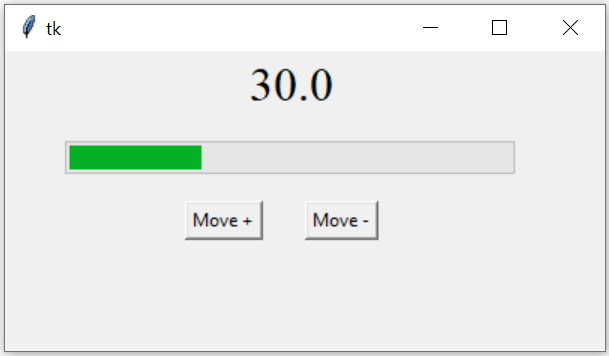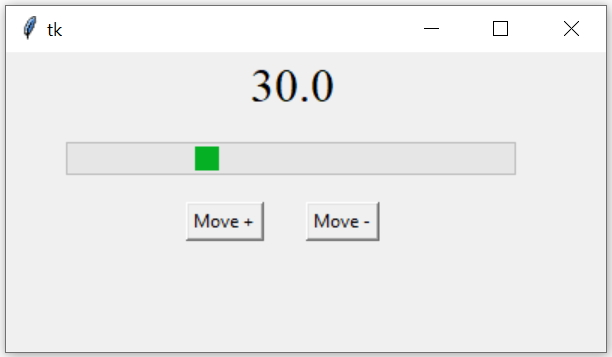# Options of Progressbar

Tkinter ProgressbarTkinter Progressbar to show status with options and methods to manage and display values

We used two buttons b1 and b2 to execute our function my_fun() on click event. This function my_fun() receives one parameter whose value is based on which button is clicked.

Inside the function the option value data is incremented or decremented based on the input parameter type and the text option of Label is updated using config().
Full code is here
``````import tkinter  as tk
from tkinter import *
from tkinter import ttk
my_w = tk.Tk()
my_w.geometry("400x200")

def my_fun(type): # update the value of progress bar
if(type=='+'):
prg1['value'] += 10 # increase value
else:
prg1['value'] -= 10 # decrease value
l1.config(text=str(prg1['value'])) #update Label

prg1 = ttk.Progressbar(my_w,orient = HORIZONTAL,
length = 300, mode = 'determinate')
prg1.place(relx=.1,rely=.3)

b1=tk.Button(my_w,text='Move +',command=lambda: my_fun('+'))
b1.place(relx=0.3,rely=0.5)

b2=tk.Button(my_w,text='Move -',command=lambda: my_fun('-'))
b2.place(relx=0.5,rely=0.5)

font1=('times',24,'normal')
l1=tk.Label(my_w,text='value here',font=font1)
l1.place(relx=0.4,rely=0.01)

my_w.mainloop()  # Keep the window open``````
We used the following options of progress bar

## modeIn the above code change the progress bar option mode to indeterminate
``````prg1 = ttk.Progressbar(my_w,orient = HORIZONTAL,
length = 300,  mode = 'indeterminate')``````

## Option value and variable

By using value option we can set and read the data of the progress bar. By using vriable option we can connect ( or update ) this data to another variable.
Check below how the value of String Variable x is used to display the data in a Label. The data associated with x is taken from the Progress bar by using the option variable

## Here is a list of all options of Progressbar

OptionsDetails
orientOrientation of the Progress bar. Values are Horizontal or Vertical
length Length of the Progress bar, (width if horizontal, height if vertical)
mode Values can be determinate OR indeterminate Check the examples
maximum Default value is 100, specifies the maximum value.
valueThe current value of the Progress bar. Can be set or read.
variableVariable can be linked to value of Progress Bar. Realtime changes ( in values ) can be captured.

Subscribe to our YouTube Channel here

## Subscribe

* indicates required
Subscribe to plus2netplus2net.com

Post your comments , suggestion , error , requirements etc here